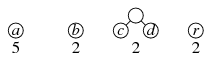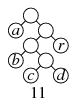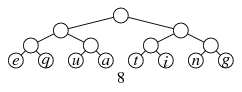Assignment 7: Huffman compression

Due: 5:00pm, Friday, October 14. Value: 40 pts. Submit to Sauron.

Huffman compression is a technique for storing data that usually results in a more condensed format than usual. The standard uncompressed technique is to use a standard pre-assigned eight-bit code to each character and store these codes sequentially in a file. (A group of eight bits, by the way, is called a byte.) The Huffman encoding is custom-built based on how often characters appear in the file, so that characters that occur more often are assigned a shorter encoding.

The technique is easiest to explain by way of example. Suppose our file consists of the following eleven letters.

We first count how often each letter occurs.

 a: 5 c: 1 r: 2 b: 2 r: 1

Then we build a binary tree according to the following technique. We begin with a forest of one-node trees, each representing a different letter. Each tree will have a frequency corresponding to the letter.Then we find the two trees that have the lowest frequency and combine them as subtrees of a root; the frequency for this tree will be the sum of the frequencies of the two subtrees.It's not important what order we put them together. We repeat this process until we have one tree left.In the case of a tie (as we had in choosing among the nodes of frequency 2 there), how we break the tie is not important.After we merge the final two trees into one, we have our Huffman tree.You may have noticed a pattern in our example — but this pattern is actually unusual. In this particular example, each combination is between the tree we've already built and a single-character node. This will usually not happen. For example, if the text were equating, where we have eight characters each appearing once, then we'd first create four trees with two letters each, and each would have a value of 2. But then we'd combine two of the trees into one of value 4, and the other two trees into another of value 4. And finally we'd combine both of these trees into a single Huffman tree.We'll return to our abracadabra tree, though. Once we have our Huffman tree, we will want to extract the encoding for it for each letter. To do this, we'll start from the root and choosing 0 when we go left and 1 when we go right. The encoding we have is as follows.

 a: 0 c: 1010 r: 11 b: 100 d: 1011

0 100 11 0 1010 0 1011 0 100 11 0

(The spaces are for presentation purposes only; they would not actually be present in the file.)

The Huffman encoding turns out to be the smallest possible prefix encoding — that is, an encoding where no character's encoding is a prefix of another character's encoding. An encoding must be a prefix encoding so that the decompression is unambiguous: If a's code is a prefix of b's code, and we want to decompress a file starting with b's code, then we wouldn't know whether the file starts with a followed by some bits representing the next letter, or whether it in fact starts with b.

(Incidentally, while Huffman encoding is the best prefix encoding, there are very different approaches to compression that perform somewhat better in practice. Some compression techniques use Huffman compression, however; the JPEG image format, for example, uses Huffman codes as one part of its very complex compression process.)

In this assignment, your job is to write a program that reads a file of characters, builds a Huffman tree, and computes the Huffman encoding for each character appearing in the file. Your program will not actually compress the file: It just computes the encoding of each letter.

The following transcript illustrates what your program should output if the user chose sample.txt containing the word abracadabra. (The first rows below are in alphabetical order, but you don't need to order the rows like this.)

```a 5 0
b 2 100
c 1 1010
d 1 1011
r 2 11
total bits: 23
```

Note that if `sample.txt` also contains an end-of-line character ('\n'), there would be another row in the table for it, and the total bits would be 29.

The output consists of two parts.

• The first lines display a table, with a line for each byte appearing in the file, giving that byte, the number of times the byte occurs, and the Huffman encoding that your program chose for the program.

You can use the following method that takes a byte and converts it to a printable string representing its value.

```// Returns a String representation of the given character.
public static String byteToString(int ch) {
if(ch == '\n') return "\\n";
if(ch == ' ')  return "\\s";
if(ch == '\t') return "\\t";
if(ch == '\\') return "\\\\";
if(Character.isISOControl((char) ch)) {
return "\\" + Integer.toOctalString((char) ch);
}
return "" + (char) ch;
}
```

The table your program prints should line up the columns in a way that looks attractive. (The above example illustrates one way.) You may print the rows in any order that is convenient.

Note that your codes for the individual letters may differ because your program may end up breaking ties differently.

• The final line displays the number of total bits that would contain the file if encoded in Huffman encoding. This is the number

c fcbc .

where fc is the number of occurrences of c in the file and bc is the number of bits in the encoding for c.

This value should be the same whatever Huffman encoding your program ends up choosing.

Run-time efficiency should not a major consideration as you complete this assignment. Of course, as always, you should strive for good, readable code.

To aid in testing, you can download both `sample.txt` and `gettysburg.txt`. The latter contains the Gettysburg address, which compresses to 6,329 bits using a Huffman encoding. (By contrast, the original file requires 11,912 bits; the compressed file is 47% smaller.)

Helper files

 `TreeNode` The `TreeNode` class we saw in class. You may modify this class. `ByteScanner` Allows easy access to the bytes of a file. You should not modify this class in this assignment.

The `ByteScanner` class allows you to open a file and read sequentially through the bytes of a file. It works much like an Iterator over bytes. It contains three methods.

`static ByteScanner promptUser()`

(Constructor method) Displays a dialog box for selecting a file, returning a `ByteScanner` corresponding to the selected file. Canceling the dialog terminates the program.

`boolean hasNext()`

Returns `true` if this file has any more bytes left in it.

`int nextByte()`

Returns the next byte in this file (between 0 and 255), and advances it to refer to the next byte instead.